# MAFS.912.G-SRT.2.4

Prove theorems about triangles. Theorems include: a line parallel to one side of a triangle divides the other two proportionally, and conversely; the Pythagorean Theorem proved using triangle similarity.
General Information
Subject Area: Mathematics
Domain-Subdomain: Geometry: Similarity, Right Triangles, & Trigonometry
Cluster: Level 3: Strategic Thinking & Complex Reasoning
Cluster: Prove theorems involving similarity. (Geometry - Major Cluster) -

Clusters should not be sorted from Major to Supporting and then taught in that order. To do so would strip the coherence of the mathematical ideas and miss the opportunity to enhance the major work of the grade with the supporting clusters.

Date of Last Rating: 02/14
Status: State Board Approved
Assessed: Yes
Test Item Specifications
assessed with:

MAFS.912.G-SRT.1.3

Sample Test Items (2)
• Test Item #: Sample Item 1
• Question:

Katherine uses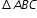, where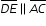to prove that a line parallel to one side of a triangle divides the other two sides proportionally. A part of her proof is shown.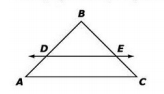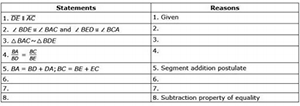Which statement completes step 8 of the proof?

• Difficulty: N/A
• Type: MC: Multiple Choice

• Test Item #: Sample Item 2
• Question: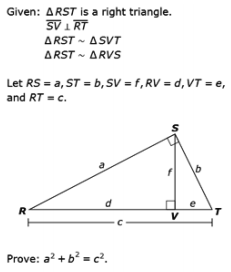An incomplete proof is shown.

Click on each blank to select a statement for row 3 and row 5 in the table.

• Difficulty: N/A
• Type: ETC: Editing Task Choice

## Related Courses

This benchmark is part of these courses.
1200400: Foundational Skills in Mathematics 9-12 (Specifically in versions: 2014 - 2015, 2015 - 2022 (current), 2022 and beyond)
1206310: Geometry (Specifically in versions: 2014 - 2015, 2015 - 2022 (current), 2022 and beyond)
1206320: Geometry Honors (Specifically in versions: 2014 - 2015, 2015 - 2022 (current), 2022 and beyond)
7912070: Access Liberal Arts Mathematics (Specifically in versions: 2014 - 2015, 2015 - 2018, 2018 - 2019, 2019 - 2022 (current), 2022 and beyond)
1206315: Geometry for Credit Recovery (Specifically in versions: 2014 - 2015, 2015 - 2022 (current), 2022 and beyond)
1207300: Liberal Arts Mathematics 1 (Specifically in versions: 2014 - 2015, 2015 - 2022 (course terminated))
7912065: Access Geometry (Specifically in versions: 2015 - 2022 (current), 2022 and beyond)

## Related Access Points

Alternate version of this benchmark for students with significant cognitive disabilities.
MAFS.912.G-SRT.2.AP.4a: Establish facts about the lengths of segments of sides of a triangle when a line parallel to one side of the triangles divides the other two sides proportionally.

## Related Resources

Vetted resources educators can use to teach the concepts and skills in this benchmark.

## Assessments

Sample 1 - High School Geometry State Interim Assessment:

This is a State Interim Assessment for 9th-12th grade.

Type: Assessment

Sample 3 - High School Geometry State Interim Assessment:

This is a State Interim Assessment for 9th-12th grade.

Type: Assessment

Sample 2 - High School Geometry State Interim Assessment:

This is a State Interim Assessment for 9th-12th grade.

Type: Assessment

## Formative Assessments

Pythagorean Theorem Proof:

Students are asked to prove the Pythagorean Theorem using similar triangles.

Type: Formative Assessment

Geometric Mean Proof:

Students are asked to prove that the length of the altitude to the hypotenuse of a right triangle is the geometric mean of the lengths of the two segments of the hypotenuse.

Type: Formative Assessment

Converse of the Triangle Proportionality Theorem:

Students are asked to prove that if a line intersecting two sides of a triangle divides those two sides proportionally, then that line is parallel to the third side.

Type: Formative Assessment

Triangle Proportionality Theorem:

Students are asked to prove that a line parallel to one side of a triangle divides the other two sides of the triangle proportionally.

Type: Formative Assessment

## Lesson Plans

Let's Prove the Pythagorean Theorem:

Students will use Triangle Similarity to derive the proof of the Pythagorean Theorem and apply this method to develop the idea of the geometric mean with respect to the relationships in right triangles.

Type: Lesson Plan

Proofs of the Pythagorean Theorem:

This lesson is intended to help you assess how well students are able to produce and evaluate geometrical proofs. In particular, this unit is intended to help you identify and assist students who have difficulties in:

• Interpreting diagrams.
• Identifying mathematical knowledge relevant to an argument.
• Linking visual and algebraic representations.
• Producing and evaluating mathematical arguments.

Type: Lesson Plan

Modeling: Rolling Cups:

This lesson unit is intended to help you assess how well students are able to choose appropriate mathematics to solve a non-routine problem, generate useful data by systematically controlling variables and develop experimental and analytical models of a physical situation.

Type: Lesson Plan

## Original Student Tutorial

Use properties, postulates, and theorems to prove a theorem about a triangle. In this interactive tutorial, you'll also learn how to prove that a line parallel to one side of a triangle divides the other two proportionally.

Type: Original Student Tutorial

## Presentation/Slideshow

The Pythagorean Theorem: Geometry’s Most Elegant Theorem:

This lesson teaches students about the history of the Pythagorean theorem, along with proofs and applications. It is geared toward high school Geometry students that have completed a year of Algebra and addresses the following national standards of the National Council of Teachers of Mathematics and the Mid-continent Research for Education and Learning: 1) Analyze characteristics and properties of two- and three-dimensional geometric shapes and develop mathematical arguments about geometric relationships; 2) Use visualization, spatial reasoning, and geometric modeling to solve problems; 3) Understand and apply basic and advanced properties of the concepts of geometry; and 4) Use the Pythagorean theorem and its converse and properties of special right triangles to solve mathematical and real-world problems. The video portion is about thirty minutes, and with breaks could be completed in 50 minutes. (You may consider completing over two classes, particularly if you want to allow more time for activities or do some of the enrichment material). These activities could be done individually, in pairs, or groups. I think 2 or 3 students is optimal. The materials required for the activities include scissors, tape, string and markers.

Type: Presentation/Slideshow

Joining two midpoints of sides of a triangle:

Using a triangle with line through it, students are tasked to show the congruent angles, and conclude if one triangle is similar to the other.

## Professional Development

Using Similarity to Prove the Pythagorean Theorem:

In the eighth grade, your students were introduced to the Pythagorean Theorem and its converse. They applied this theorem to a variety of real-world and mathematical problems to find the lengths of sides of right triangles. Now that your students understand concepts related to similarity, they are able to appreciate a simple proof of the Pythagorean Theorem. The focus of this tutorial is on describing and explaining a proof of the Pythagorean Theorem using similar triangles.

Type: Professional Development

## Tutorials

Bhaskara's Proof of the Pythagorean Theorem:

This video demonstrates Bhaskara's proof of the Pythagorean Theorem.

Type: Tutorial

Another Pythagorean Theorem Proof:

This video visually proves the Pythagorean Theorem using triangles and parallelograms.

Type: Tutorial

Pythagorean Theorem Proof Using Similar Triangles:

This video shows a proof of the Pythagorean Theorem using similar triangles.

Type: Tutorial

## Video/Audio/Animation

Annotated Proof of the Pythagorean Theorem :

This resource gives an animated and then annotated proof of the Pythagorean Theorem.

Type: Video/Audio/Animation

## Virtual Manipulative

Demonstrate the Pythagorean Theorem:

Representation to illustrate the Pythagorean Theorem.

Type: Virtual Manipulative

## MFAS Formative Assessments

Converse of the Triangle Proportionality Theorem:

Students are asked to prove that if a line intersecting two sides of a triangle divides those two sides proportionally, then that line is parallel to the third side.

Geometric Mean Proof:

Students are asked to prove that the length of the altitude to the hypotenuse of a right triangle is the geometric mean of the lengths of the two segments of the hypotenuse.

Pythagorean Theorem Proof:

Students are asked to prove the Pythagorean Theorem using similar triangles.

Triangle Proportionality Theorem:

Students are asked to prove that a line parallel to one side of a triangle divides the other two sides of the triangle proportionally.

## Original Student Tutorials Mathematics - Grades 9-12

Use properties, postulates, and theorems to prove a theorem about a triangle. In this interactive tutorial, you'll also learn how to prove that a line parallel to one side of a triangle divides the other two proportionally.

## Student Resources

Vetted resources students can use to learn the concepts and skills in this benchmark.

## Original Student Tutorial

Use properties, postulates, and theorems to prove a theorem about a triangle. In this interactive tutorial, you'll also learn how to prove that a line parallel to one side of a triangle divides the other two proportionally.

Type: Original Student Tutorial

## Presentation/Slideshow

The Pythagorean Theorem: Geometry’s Most Elegant Theorem:

This lesson teaches students about the history of the Pythagorean theorem, along with proofs and applications. It is geared toward high school Geometry students that have completed a year of Algebra and addresses the following national standards of the National Council of Teachers of Mathematics and the Mid-continent Research for Education and Learning: 1) Analyze characteristics and properties of two- and three-dimensional geometric shapes and develop mathematical arguments about geometric relationships; 2) Use visualization, spatial reasoning, and geometric modeling to solve problems; 3) Understand and apply basic and advanced properties of the concepts of geometry; and 4) Use the Pythagorean theorem and its converse and properties of special right triangles to solve mathematical and real-world problems. The video portion is about thirty minutes, and with breaks could be completed in 50 minutes. (You may consider completing over two classes, particularly if you want to allow more time for activities or do some of the enrichment material). These activities could be done individually, in pairs, or groups. I think 2 or 3 students is optimal. The materials required for the activities include scissors, tape, string and markers.

Type: Presentation/Slideshow

Joining two midpoints of sides of a triangle:

Using a triangle with line through it, students are tasked to show the congruent angles, and conclude if one triangle is similar to the other.

## Tutorials

Bhaskara's Proof of the Pythagorean Theorem:

This video demonstrates Bhaskara's proof of the Pythagorean Theorem.

Type: Tutorial

Another Pythagorean Theorem Proof:

This video visually proves the Pythagorean Theorem using triangles and parallelograms.

Type: Tutorial

Pythagorean Theorem Proof Using Similar Triangles:

This video shows a proof of the Pythagorean Theorem using similar triangles.

Type: Tutorial

## Video/Audio/Animation

Annotated Proof of the Pythagorean Theorem :

This resource gives an animated and then annotated proof of the Pythagorean Theorem.

Type: Video/Audio/Animation

## Parent Resources

Vetted resources caregivers can use to help students learn the concepts and skills in this benchmark.